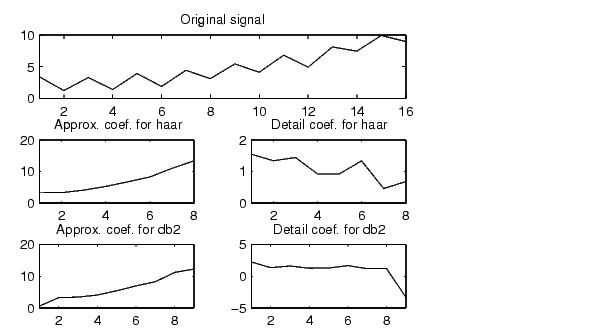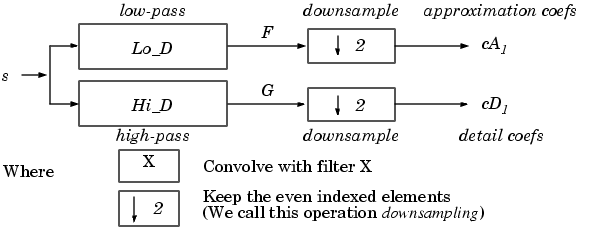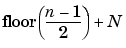Wavelet Toolboxdwt

Single-level discrete 1-D wavelet transform

Syntax

• ```[cA,cD] = dwt(X,'`wname`')
[cA,cD] = dwt(X,'`wname`'`,`'`mode`'`,MODE`)
[cA,cD] = dwt(X,Lo_D,Hi_D)
[cA,cD] = dwt(X,Lo_D,Hi_D`,`'`mode`'`,MODE`)
```

Description

The `dwt` command performs a single-level one-dimensional wavelet decomposition with respect to either a particular wavelet ('`wname`', see `wfilters` for more information) or particular wavelet decomposition filters (`Lo_D` and `Hi_D`) that you specify.

`[cA,cD] = dwt(X,`'`wname`'`)` computes the approximation coefficients vector `cA` and detail coefficients vector `cD`, obtained by a wavelet decomposition of the vector `X`. The string '`wname`' contains the wavelet name.

`[cA,cD] = dwt(X,Lo_D,Hi_D)` computes the wavelet decomposition as above, given these filters as input:

• `Lo_D` is the decomposition low-pass filter.
• `Hi_D` is the decomposition high-pass filter.

`Lo_D` and `Hi_D` must be the same length.

Let `lx` = the length of `X` and `lf` = the length of the filters `Lo_D` and `Hi_D`; then `length(cA) = length(cD) = la` where `la = ceil(lx/2)`, if the DWT extension mode is set to periodization. For the other extension modes,` la` = `floor(lx+lf-1)/2)`.

For more information about the different Discrete Wavelet Transform extension modes, see `dwtmode`.

`[cA,cD] = dwt(...,`'`mode`'`,MODE)` computes the wavelet decomposition with the extension mode `MODE` that you specify. `MODE` is a string containing the desired extension mode.

Example:

• ````[cA,cD] = dwt(x,`'`db1`'`,`'`mode`'`,`'`sym`'```);
``````

Examples

• ```% The current extension mode is zero-padding (see `dwtmode`).

% Construct elementary original one-dimensional signal.
randn('seed',531316785)
s = 2 + kron(ones(1,8),[1 -1]) + ...
((1:16).^2)/32 + 0.2*randn(1,16);

% Perform single-level discrete wavelet transform of s by haar.
[ca1,cd1] = dwt(s,'haar');
subplot(311); plot(s); title('Original signal');
subplot(323); plot(ca1); title('Approx. coef. for haar');
subplot(324); plot(cd1); title('Detail coef. for haar');

% For a given wavelet, compute the two associated decomposition
% filters and compute approximation and detail coefficients
% using directly the filters.
[Lo_D,Hi_D] = wfilters('haar','d');
[ca1,cd1] = dwt(s,Lo_D,Hi_D);

% Perform single-level discrete wavelet transform of s by db2
% and observe edge effects for last coefficients.
% These extra coefficients are only used to ensure exact
% global reconstruction.
[ca2,cd2] = dwt(s,'db2');
subplot(325); plot(ca2); title('Approx. coef. for db2');
subplot(326); plot(cd2); title('Detail coef. for db2');

% Editing some graphical properties,
% the following figure is generated.```

Algorithm

Starting from a signal s, two sets of coefficients are computed: approximation coefficients CA1, and detail coefficients CD1. These vectors are obtained by convolving s with the low-pass filter Lo_D for approximation and with the high-pass filter Hi_D for detail, followed by dyadic decimation.

More precisely, the first step isThe length of each filter is equal to 2N. If n = `length`(s), the signals F and G are of length n + 2N - 1, and then the coefficients CA1 and CD1 are of length

•To deal with signal-end effects involved by a convolution-based algorithm, a global variable managed by `dwtmode` is used. This variable defines the kind of signal extension mode used. The possible options include zero-padding (used in the previous example) and symmetric extension, which is the default mode.

```dwtmode, idwt, wavedec, waveinfo ```dtree dwt2